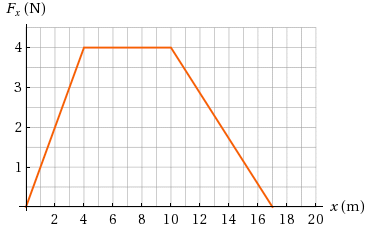Question
10 views

A object of mass 3.00 kg is subject to a force Fx that varies with position as in the figure below.

If the object has a speed of 0.400 m/s at x = 0, find its speed at x = 4.00 m and its speed at x = 17.0 m

speed at x = 4.00 m = ? m/s

speed at x = 17.0 m= ? m/shelp_outlineImage TranscriptioncloseF, (N) 4 3 x (m) 16 18 20 10 12 14 2 4 6 8 fullscreen
check_circle

Step 1

Given,

Step 2

Graph for given figure has been shown below,

Step 3

Part (a):

As the graph given is F-x graph so the area of given gr...

### Want to see the full answer?

See Solution

#### Want to see this answer and more?

Solutions are written by subject experts who are available 24/7. Questions are typically answered within 1 hour.*

See Solution
*Response times may vary by subject and question.
Tagged in

### Work,Power and Energy